# Riemann curvature tensor of Levi-Civita connection

{{tensoroftype|(1,3)}

## Definition

### As a (1,3)-tensor

Let$M$ be a connected differential manifold and$g$ be a Riemannian metric or pseudo-Riemannian metric on$M$. Let$\nabla$ denote the Levi-Civita connection of$g$. The Riemann curvature tensor for the Riemannian metric is defined as the Riemann curvature tensor of the Levi-Civita connection, viz: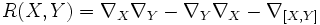$R(X,Y) = \nabla_X \nabla_Y - \nabla_Y \nabla_X - \nabla_{[X,Y]}$

Since the Levi-Civita connection is a linear connection,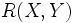$R(X,Y)$ is a linear map from the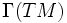$\Gamma(TM)$ to$\Gamma(TM)$. Thus, we can view the Riemann curvature tensor as a map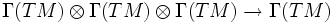$\Gamma(TM) \otimes \Gamma(TM) \otimes \Gamma(TM) \to \Gamma(TM)$ given as: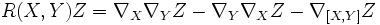$R(X,Y)Z = \nabla_X \nabla_YZ - \nabla_Y \nabla_XZ - \nabla_{[X,Y]}Z$

That this is a tensor follows from the fact that the Riemann curvature tensor is a tensor. For full proof, refer: Curvature is tensorial

### As a (0,4)-tensor

A Riemannian metric or pseudo-Riemannian metric on a differential manifold brings about an isomorphism between its tangent bundle and its cotangent bundle, sending$v \in \Gamma(TM)$ to the element$w \mapsto g(v,w)$ in the cotangent bundle. Thus any$(p,q)$ tensor can be transformed to a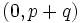$(0,p+q)$-tensor. In particular the Riemann curvature tensor can be transformed to a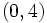$(0,4)$-tensor, explicitly described as: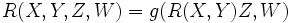$R(X,Y,Z,W) = g(R(X,Y)Z,W)$

## Facts

### Tensoriality in all variables

The Riemann curvature tensor of the Levi-Civita connection is tensorial in all the variables. This follows from the fact that the general Riemann curvature tensor is tensorial in all the three variables. For full proof, refer: Tensoriality of Riemann curvature tensor

### Antisymmetry in the first two variables

The antisymmetry in the first two variables again follows from the fact that the Riemann curvature tensor is alternating in the first two variables. For full proof, refer: Antisymmetry of Riemann curvature tensor

### Antisymmetry in the last two variables

This is a somewhat surprising fact:$R(X,Y,Z,W) = -R(X,Y,W,Z)$

Another way of saying this is that the adjoint of the operator$R(X,Y)$ is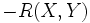$-R(X,Y)$. For full proof, refer: Curvature is antisymmetric in last two variables

### Symmetry in the two pairs

From the fact that the Riemann curvature tensor is antisymmetric in both the first two and the last two variables, we can view it as a map: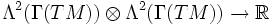$\Lambda^2(\Gamma(TM)) \otimes \Lambda^2(\Gamma(TM)) \to \R$

It turns out that the map is symmetric in the two arguments. In other words, it can be viewed as an element of$\operatorname{Sym}^2(\Lambda^2(\Gamma(TM)))^*$.

More explicitly: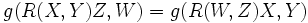$g(R(X,Y)Z,W) = g(R(W,Z)X,Y)$

Note that$X$ gets interchanged with$W$ and <amth>Y[/itex] with$Z$.

### First Bianchi identity

Further information: First Bianchi identity

Also known as the algebraic Bianchi identity, this identity holds not just for the Levi-Civita connection, but more generally, for any torsion-free linear connection over the differential manifold. It states that: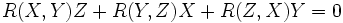$R(X,Y)Z + R(Y,Z)X + R(Z,X)Y = 0$

### Second Bianchi identity

Further information: Second Bianchi identity

In this case, the identity can be written as:$(\nabla_XR)(Y,Z) + (\nabla_YR)(Z,X) + (\nabla_ZR)(X,Y) = 0$